Se muestran los artículos pertenecientes al tema 4ESO FQ (10th gr Science) 2018-2019.

## 4ESO FQ English 2018-2019. Unit 5 WORK AND ENERGY. Article 7.

Publicado: 26/04/2019 06:36 por easyfisquim en 4ESO FQ (10th gr Science) 2018-2019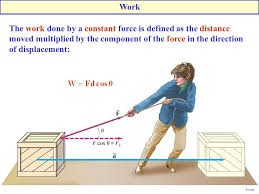WORK AND ENERGY

- Website to have a general view and study of this lesson 5 about Work and energy.

+ What is this lesson about and what does work and energy lesson study? :

When a force acts upon an object to cause a displacement of the object, it is said that work was done upon the object. There are three key ingredients to work - force, displacement, and cause. In order for a force to qualify as having done work on an object, there must be a displacement and the force must cause the displacement. There are several good examples of work that can be observed in everyday life - a horse pulling a plow through the field, a father pushing a grocery cart down the aisle of a grocery store, a freshman lifting a backpack full of books upon her shoulder, a weightlifter lifting a barbell above his head, an Olympian launching the shot-put, etc. In each case described here there is a force exerted upon an object to cause that object to be displaced.

In this unit, an entirely different model will be used to analyze the motion of objects. Motion will be approached from the perspective of work and energy. The effect that work has upon the energy of an object (or system of objects) will be investigated; the resulting velocity and/or height of the object can then be predicted from energy information. In order to understand this work-energy approach to the analysis of motion, it is important to first have a solid understanding of a few basic terms. Thus, Lesson 1 of this unit will focus on the definitions and meanings of such terms as work, mechanical energy, potential energy, kinetic energy, and power.

+ Work and energy definition VIDEO                              Go there !!

+ Word definition activity and problems to solve :      Page 1       Page 2       Page 3       Page 4

## 4ESO FQ English 2018-2019. Unit 4 FLUID STATICS (FORCES). Article 6.

Publicado: 04/04/2019 10:05 por easyfisquim en 4ESO FQ (10th gr Science) 2018-2019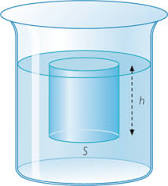FLUID STATICS

- Website to have a general view and study of this lesson 4 about Fluid statics.

+ What is this lesson about and what does fluid statics study? :

Fluid statics is the branch of fluid mechanics that studies incompressible fluids at rest. It encompasses the study of the conditions under which fluids are at rest in stable equilibrium as opposed to fluid dynamics, the study of fluids in motion.

+ Prelude to Fluid Statics :

What exactly is a fluid? Can we understand fluids with the laws already presented, or will new laws emerge from their study? The physical characteristics of static or stationary fluids and some of the laws that govern their behavior are the topics of this chapter.

* A fluid is a state of matter that yields to sideways or shearing forces. Liquids and gases are both fluids.

+ Fluids vocabulary :                   Fluids dynamics gap text

+  What Is a Fluid?                Go there !!

Liquids and gases are considered to be fluids because they yield to shearing forces, whereas solids resist them. Note that the extent to which fluids yield to shearing forces (and hence flow easily and quickly) depends on a quantity called the viscosity.

+ Density                 Go there !!    Check and copy the definition of density!!

Which weighs more, a ton of feathers or a ton of bricks? This old riddle plays with the
distinction between mass and density. A ton is a ton, of course; but bricks have much
greater density than feathers, and so we are tempted to think of them as heavier.

* Density exercise : CALCULATING THE MASS OF A
RESERVOIR FROM ITS VOLUME.

A reservoir has a surface area of 50km2 and an average depth of 40.0m. What mass of                                 water is held behind the dam?

Problem solved :  FOTO

* Go now to this LINK and produce a table in which to organize several
substances according to their density indicating which ones will float or sink.
Write down their density value and units and beside it say if it will float or
not just comparing them to the water density ( 1 g/ml ).

- Density problems to work out.  Just try them  ( you can check the solutions there).
( Please try to work out the problems by yourself first. If you finally
need help then check the answers given ).

- Pressure is the force per unit perpendicular area over which the force is applied.     In equation form, pressure is defined as :
P = F/A     Pressure equals force divided by surface or area.

F = P.A

The SI unit of pressure is pascal and 1 Pa=1 N/m2.

+ Lets go to this LINK to learn how important pressure is when considering fluids.

+ ACTIVITY FOR FRIDAY ON THE COMPUTERS :

*Check these two websites  (  WEB1  and  WEB2  ) and make a powerpoint or a word file just organizing and summarizing the different kind of pressures we can find in nature. Make a table or a chart organizing them while explaining their most important features. When you are done (finished) send your work to your teacher's email.

## 4ESO FQ English 2018-2019. Unit 3 DYNAMICS (FORCES). Article 5.

Publicado: 22/03/2019 10:02 por easyfisquim en 4ESO FQ (10th gr Science) 2018-2019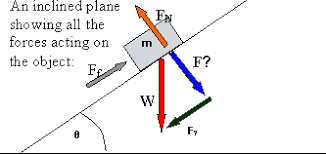DYNAMICS.

- You already know what Dynamic is from what you studied before about forces lesson. But you have anyway a reminder here :

+ Dynamics, branch of physical science and subdivision of mechanics that is concerned with the motion of material objects in relation to the physical factors that affect them: force, mass, momentum, energy. Newtons laws of motion are directly related to Dynamics and, even more, they explain it.

- MASS AND WEIGHT :

* Activity 1.32  :   Gravity and weight.                                          ( Monday )

*   These are the questions to answer  looking up the following links :

1.32.a   GRAVITY, MASS AND WEIGHT. ( slides )

1.32.b   MASS AND WEIGHT.     (hyperphysics website with definitions and equations).

1.32.c   GRAVITY.

Check your weight on other planets.

Here you can work out mass, gravity or weight.

When you finish answering all the questions.           ( 20 points ).

+ Do this PROBLEM about finding Jupiters gravity from knowing a persons mass and his/her weight.

> When attraction between Earth and an object is considered, Newtons gravitation equation becomes like this :

+ NEWTONS GRAVITATION EQUATION PROBLEMS.       Just try them !!

+ Here you have a problem about Gravitational attraction force  ( wording pic and then a solution pic ) in
which one you have to solve for another variable different from the attraction force F.

+ At the following website you can check all whatever you want about GRAVITATION !!   ( Keplers laws included ).

+ VIDEO 1  easy explained the 3 Keplers laws.
+ VIDEO 2   easy explained the 3 Keplers laws.
+ VIDEO 3  easy explained the 3 Keplers laws.
+ VIDEO 4  easy explained the 3 Keplers laws.
+ VIDEO 5  even one more video explaining Keplers laws.
+ Right  HERE  you can find a page with an easy way to learn and understand   Keplers laws.

+ Lets try now the exercises 1 and 3 from the following website about Keplers laws.
+ Lets try a few more problems about solving and using Keples laws.

* Activity 1.34  :   Reviewing Mass and gravity.                                          ( Monday )

1.34.a   Pics of earth, gravity and weight to make the poster.         ( 10 points ).

1.34.c   Fun way to review forces.              ( 5 points ).

Copy this outline on a sheet in order to review some of the forces studied.

LINK 2  ( Weight , mass , gravity ).

* Activity 1.33  :   Gravity and weight test.                                     ( Tuesday )

GRAVITY , MASS AND WEIGHT TEST.                                ( 10  points ).

Newtons laws quizzes.

-  Gravity text to be worked on class :  Page 1   Page 2   Page 3   Page 4

-  Gravity cards for a talking activity :

- INCLINED PLANE ( advanced extra class ) :

- An inclined plane, also known as a ramp, is a flat supporting surface tilted at an angle, with one end higher than the other, used as an aid for raising or lowering a load. The inclined plane is one of the six classical simple machines defined by Renaissance scientists.

- Now it is time for you to achieve an exercise like that. Just try doing the
following exercise :

+ Wording exercise 1

Find the components of a force F whose magnitude is 20 N and its direction is
defined by angle θ = 30° made by the force and the positive x-axis as shown below.

+ Wording exercise 2

Find the components of a force whose magnitude is 50 N and its direction is given by                           angle α = 25° made with the negative y-axis as shown in the diagram.

+ You can find the solutions and explanations about the way to achieve
them HERE.

+ HERE   ,   You can find more exercises of the same kind.

## 4ESO FQ English 2018-2019. Unit 2 FORCES. Article 4.

Publicado: 10/12/2018 23:17 por easyfisquim en 4ESO FQ (10th gr Science) 2018-2019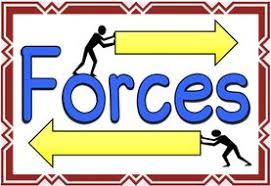- Lets check these first links in order to find out what a force is and which is the
best way to explain it by writting :

e) Forces, motion and gravity.

1- What is a foce?

2- How can be forces measured?.

3- What is the connection between forces and acceleration?.

+ Forces LINK 1 ( forces problems about concurrent and non concurrent forces )

+ Forces LINK 2 (examples of kind of forces )

+ Forces LINK 4 ( normal force and equilibrium force )

+ Forces LINK 5 ( weight, mass and gravity force )

+ Forces LINK 6 ( friction force and video about Newtons laws )

- Forces, vectors and Newtons laws worksheets and peer  talking assessment
rubric :

In physics, concurrent forces are defined as forces that pass through a
common point. In other words, a concurrent force system is a set of two or
more forces whose lines of action intersect at a point at the same time.

* Concurrent forces image example :     FOTO

* Concurrent forces Video example exercise :   VIDEO

* Net Force calculation on concurrent forces exercises with the
same and opposite direction :   LINK

* Concurrent forces exercises :  Page 1        Page 2
And the exercises solutions :   Page 3        Page 4

* Tug of war game, concurrent forces exercise example :  FOTO

* Parallelogram method to get net Force :  FOTO 1     FOTO 2

* Parallelogram method exercises 1 i 2 :  FOTO 1      FOTO 2

* Parallelogram method for balanced forces in equilibrium :  FOTO

* Concurrent forces ex1 : FOTO

* Concurrent forces ex3 : FOTO

* Concurrent forces SCALE ex5 : FOTO

* Exam from past year about concurrent and non concurrent forces :

(At the following pic you find the answers to the exercises from this exam) : LINK

* More complicated exercises examples related to concurrent forces
when involving coplanar ( 2-dimensional ) forces. Examples about
two tugboats towing a cargo ship (exercise 1) :    LINK

- Non concurrent forces :  LINK

A couple is an example of a non-concurrent force because its
line of action does not intersect at one point.

* Non concurrent forces image example :       FOTO

* This is a great link where you can find and learn a lot about
working out resultant net forces on non concurrent like and
unlike parallel forces systems :   LINK

** And here you can check it but in a pdf file :  PDF

- Whats a TORQUE ?  A TORQUE is  ( read also this website )

- Website with problems having a TORQUE  involved :  Website

- You can follow a nice explanation video about NON CONCURRENT PARALLEL FORCES separated certain distance :

* At this link you can look up how to work out the resultant of of parallel coplanar forces :   LINK

+ And at this website you have a very clear explanation about an example of two unlike parallel forces
exercise and how to find out the net resultant force and where its point of application is plot :

-  After youve practiced and watched the different videos, try to do the following exercise by your own. Check previous videos as many times as you need to develope the exercise by yourself. In case you cannot achieve the problems solution you have the problem explained at the video below :

> Exercise :

A 15kg metal bar 6m long has two objects hanged at the opposite ends of the bar. At the left end of the bar hangs an 20kg object and at the right end of the bar the second 30kg object. Find where exactly the net force must be placed in order not to let the system fall. Work out the value of the net or balance force, the exact spot it must be placed, the direction and if it is like or unlike to the forces involved initially.

+ Now try by yourself the following exercises about non concurrent parallel forces:

Wording exercise 1 :   FOTO  ( like forces )
Solution exercise 1:     FOTO

Wording exercise 2 :    FOTO( unlike forces )
Solution exercise 2 :    FOTO

+ Activity about first Newtons law :  Watch these VIDEO1 and VIDEO2
then do the following :

a) Whats the first Newtons law and what does it talk about? Write the answer
down on your notebook. Make a Word file to do it if you prefer.

b) Draw an outline or a design or an example in which you could explain the
first Newtons law is being applied.

+ Activity about second Newtons law :

a) Read what this website has to say about second Newtons law and
summarize the most important at your notebook or on a Word file.

b) Go to this SIMULATOR website and check how second Newtons law
can change objects motion.

+ Activity about third Newtons law :

third Newtons law is and what it says. You can achieve the activity on
a Word file too if you prefer.

b) Go to this LINK and give an answer to every example and draw the
action and reaction forces acting in each one.

+ NET FORCE and 2nd Newtons law : Go to this link.

+ RESISTIVE OR FRICTION FORCE and 2nd Newtons law :

+ Next check this fantastic webpage about 2nd Newtons law and all the main
issues involved. Youll find drawings, exercises, examples, definitions, it is
just great!! , it also explains friction force with exemples :

+ NEWTONSS LAWS GLIMPSE :   FOTO   ( text with gaps to fill out )

+ Specific ACTIVITIES ABOUT 2nd NEWTONS LAW :

* Steps to follow to work out 2nd Newtons law problema with friction force
involved. Check it to see how to figure out this kind of problems :  FOTO

* 2nd Newtons law leaflet with exercises to practice :   PDF

+ Solution of the 6 problems from the last leaflet about 2nd law :

FOTO 1     FOTO 2     FOTO 3

* At this link you can find explanations, from easy ones to more complicated ones,
about how to figure out resultant forces within different force systems. You can
also have there a wide variety of problems with solutions :   LINK
( At the end of that website youll find a link with problems ).

+ This is a nicely done webpage from BBC in order to revew Newtons main
three laws about motion. You have there explanations, exemples, videos,
graphs, outlines, working out problems and much more. Just check it!! :

- HOOKEs LAW :

+ Whatch these videos now :  VIDEO 1   and   VIDEO 2  about Hookes law.

Pay attention now to the following PIC and the related GRAPH showing a
spring stretching and then answer the following questions and exercises.

+ Question 1 : Whats happening at the PICs image?. Explain it and
write it down.

+ Question 2 : Imagine that each weight that is added at the PICs
image is about 7N. Write down the formula you should
use you explain the Force the spring is doing. Afterwards
figure out the spring stiffness constant using that formula
and the values of displacement and force that are
displayed at the PIC.

+ Question 3 : Take a look again at this Hookes spring law GRAPH and
find the spring stiffness constant and also the stretching
spring will take when a force of 3N is applied.

+ Question 4 :  Do exercise 2 from page 43.

* Forces Lesson multiple choice TEST

- Forces in Architecture :

Architecture and how they are spread out within the building structure.

architecture and the different ways that exist to spread out forces usind
different kind of buttress and flying buttress ( arbotante ).

Once you have already checked, read and looked up all this material
and these websites, just make a presentation about explaining and
showing how importants forces have been in architecture throughout
history and the methods and improvements that have been achieved
to make possible to buid bigger and greater buildings. You can make a

## 4ESO FQ English 2018-2019. Unit 1 MOTION. Article 3.

Publicado: 24/10/2018 02:47 por easyfisquim en 4ESO FQ (10th gr Science) 2018-2019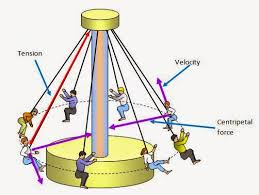UNIT 1 :    MOTION    ( Lesson 3)

SECTION 3 , LESSON 1 ( Gravity and free falling motion).

(  Free falling object motion or dropping motion  ,  circular motion).

-  First of all lets watch a video about what free fall vertical motion is.

*After watching that video, what do you think are the most important issues
we have to have on account when approaching and facing a free fall motion
exercise or situation?.
SCAFF 7d :  Fill out the chart .

+Next youll find the chart filled in, to check it and correct your answers and
the way to fill it out. It Will be posted once we achieve yet the activity in class.

-  Link 1:  Free falling motion introduction website.  Motions details and gravity.
-  Link 2:  Read here what GRAVITY is and what gravitys acceleration is.

-  Link 3:  This website has really remarkable information, solved exercises,

- Lets watch this video about how to solve a one dimensión MRUA free fall motion
( upwards thrown object motion ) exercise and after watching it
were gonna work it out again by ourselves.
This would be the wording about the problem this teacher is figuring out :

A kid is placed 20m high from the ground and throws an object upwards with a
velocity of 15m/s. Find out the time the object takes to fall and  hit the ground
and the final velocity it takes just before hitting the ground.

- Lets just do the same exersice as the previous one above but this time the kid
is throwing the object from the same high at the same speed but to the down
direction, to hit the ground directly.  Watch the video now in class or at home
(follow your teachers directions) and we do it later on the board.

SCAFF 8 :
-  Pic Activity   :    Check this pic your teachers taken showing the equations
between MRUA (uarm) and free falling motion. Write on your
notebook what they both have in common and why they have
something different. Youll need to read the links 1, 2 and 3 from
above of this lesson to know well what free falling motion is

-  At this point we do exercises 11 and 12 on page 27 from your students book.

-  Lets check : Upward thrown object motion exercise example 8 on page 28.

-  After checking the example, lets try this one!! :

A person standing on the edge of a high cliff throws a rock straight up with an initial velocity of 13.0 m/s. The rock misses the edge of the cliff as it falls back to earth. Calculate the position and velocity of the rock 1.00 s, 2.00 s, and 3.00 s after it is thrown, neglecting the effects of air resistance.
sol : within LINK 3 above

- Watch the following pic about Hulk, Spiderman and gravity and have a small
talk in groups or in pairs to give your opinion about the studied motions involved.

-  Now we do exercises 13, 14 and 15 on page 28 from your book.

solutions :   ex 13     ,     ex 14     ,     ex 15

-  Link  : Link from the last school years with free falling motion exercises and
examples and videos helping solving this kind of problems.

-  Link :  Free fall motion complex problem to solve, and solution provided.

*  Take a look to this cartoon and discuss whats involved in it

- To review the lesson you can try doing the following exercises on page 35 from
your book. You are also given the solutions at the following links :

UNIFORM CIRCULAR MOTION.

-  Link 1a:    Uniform circular motion video to start.

-  Link 1b:    Visualize circular motion agains constant acceleration motion.

-  Link 2:    Circular motion main equation and information.

-  Link 3:    Circular motion teachers website with physics related to circular
motion.

-  Link 4 :     Circular motion experience 1 done by last years former students.

* Choose one of the following experiences to make:

-  Link 5a:      Uniform circular motion experience 2 to achieve.
-  Link 5b:      Uniform circular motion experience 3 to achieve.
You can also check the same experience as 5b here in 5c.

+  How to transform or convert degrees to radians and vice versa :

factors from degrees to radians and back.

- Once youve checked all the materials from this blog and you have already organized your 3 or 4 people group, share responsabilities among your groups members in order to write a general outline for giving a speech later showing your contents about uniform circular motion, linear velocity, angular velocity, differences among them and conclusions. Dont forget to give experimental data and examples to substantiate your reasons.

* If needed you can get help to collect vocabulary and structures to write your text from the quizz and all other materials provided at the blog.

- You do now exercises 16, 17 and 18 on page 30.

solutions :           ex 16 and 17               ex18

- Circular motion exercise from the blackboard .  Wording :

Some friends are skating around in circles holding by their hands in line. A girl
at the opposite end of the line is 12m far away from the center of the circle they
are spinning around. One girls friend is closer to the center spinning with an
angular velocity of Pi/2 rad/s and a linear velocity of 4m/s.

Find the linear velocity the girl shows at the Edge of the skating line in a certain
momento and the distance the boy is placed from the center of the circle.

*Check the solution here on these two pics :

- Make a lesson summary like this one appearing on page 31. Do it in english
language and try to use your own words.

ORAL PRESENTATION. MOTION ISSUE SPEECH.

- And now you can check on these files the steps to be followed in order to make
and design an oral presentation about one specific motion lesson issue left up
to you.  On the first file you will find some help, advises and rules to write your
presentation and some important aspects to keep in mind when you make your
speech to the audience. At the second one you are provided by information and
tips in order to assess your peers presentations :

- Students pics for the MOTION ISSUE speeches :

Diana :   Foto1   Foto 2   Foto 3   Foto 4

Raúl :     Foto1

Wiam :   Foto1

Marco :   Foto1

Nicole:    Foto1

Anna:     Foto1

Dylan:    Foto1

Aida:      PowerPoint 1

## 4ESO FQ English 2018-2019. Unit 1 MOTION. Article 2.

Publicado: 07/10/2018 19:28 por easyfisquim en 4ESO FQ (10th gr Science) 2018-2019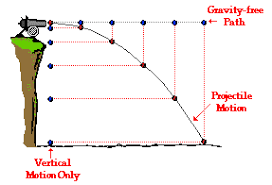UNIT 1 :    MOTION

SECTION 2 , LESSON 1,  MRUA.
( Moviment rectilini uniformement accelerat, Constant or uniform acceleration motion ).

-  Converting units with conversion factors review.

( Do the unit changes suggested in class ).
( Corrections of the exercises suggested ).

- Read the following description about whats the difference between velocity and acceleration, check also the corresponding equations and try to summarize the main issues at your notebook. Write it down if you agree with it too  :

The difference between velocity and acceleration can be drawn clearly on the following grounds:

The velocity of an object refers to the speed in a specific direction.
Velocity is nothing but the rate of change of displacement. On the other hand, acceleration is the rate of change of velocity  with respect to time.

SCAFF 6 : - Traffic accident pic and talking.
File with scaffolding material to talk about the pic.

- Example 4 pag 23.

- Make and achieve exercises 8 and 9 on page 23.         Solutions :          ex8             ex9

Teachers paper with first MRUA equations proof.   X=Xo+Vo.t+1/2a.t^2

* Second important equation demonstration.    V^2-Vo^2=2.a.(X-Xo)

- Example 5 page 24.

* Useful links to check to review constant acceleration motion lesson :

- MRUA link 1  ( Video explaining MRUA and main MRUA equations and graphs ).

- MRUA link 2  ( Several links and videos working on MRUA main issues ).

- MRUA link 3  ( Acceleration and gravity, linked equations, exercises and examples ).

- Exercises 43, 44 and 56 and 56end pag 34 and 35.

- Homework to do for next monday :  ex 45, 46 and 47 on page 34.

- Exercise MRUA to achieve , quadratic equation to get time.

SCAFF 7 :- MRUA graphs text to fill out.  1 , 2 and 3.  ------   Solutions :  1  ,  2   and   3.

-  Lets do this one now:       Exercise to do about working out the time to go over certain
distance in MRUA.

A car starts its movement with a u.r.a.m (MRUA) motion. Its showing an acceleration of
12m/s2.  How much time will it take to cover or go over 625m?.

Solution:  Check the solution HERE

+ In this Link you have a great help with a website provided in order to be able to create any kind of graph
you need to show your data ( bar graphs, line graphs, pie charts...).  Just choose your desing, color,
back ground and introduce your data and the labels you want to appear on the chart :

- Homework  :

Look over the   Example 6 pag 26.  where several stages from a MRUA motion are described.
Your task for next monday is to prepare an outline or a text using similar explanations but in english to
describe whats happening at the graph but making use of your own words and expressions.
Then copy the graph, check the scaffolding  material provided on the last lesson to write your sentences
and organize the paragraphs to explain what is shown at the graph and what the numerical calculations
and results mean. Prepare a single separated sheet from your workbook to hand it in to your teacher.

SCAFF 7b : Check this FILE to get some help to do the homework activity above.

SCAFF 7c :  Check this FILE to fill out 2 grids which will help you a lot to write your essay or writing

- Do now exercises 51, 52 and 53 on page 34 and later exercise 57 on page 35.

Solutions :           ex 51 part1  ;   ex 51 part 2

ex 52 and 53 on page 34

ex 57 on page 35

## 4ESO FQ English 2018-2019. Unit 1 MOTION. Article 1.

Publicado: 13/09/2018 19:57 por easyfisquim en 4ESO FQ (10th gr Science) 2018-2019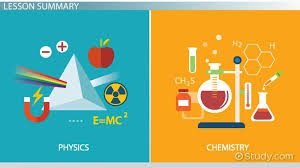UNIT 1 :    MOTION

SECTION 1 , LESSON 1,  MRU.
( Moviment rectilini uniforme, Linear straight uniform motion ).

* Exercises wordings of whole UNIT 1  :    1      2      3      4      5      6      7

8      9    10    11    12

> Solutions to exercices 30 to 3233 , 34 and 35.

> Solution of ex3 from section 2 :   solution

-  Subject introduction, units, class organization and working class issues.

PHYSICS CLASSROOM WEBSITE.

SCAFF 1 :  This file provides you with enough vocabulary to explain what
is this subject going about. Use it and write down a couple of

- How to say "entregar un trabajo" :

to turn in or hand in something, such as a school assignment, paper, etc., to someone. They were told to pass their papers in to the teacher.

- How to say "pasa estos papeles o hojas a los demás" :

Speed :  Just as distance and displacement have distinctly different meanings (despite their similarities), so do speed and velocity. Speed is a scalar quantity that refers to "how fast an object is moving." Speed can be thought of as the rate at which an object covers distance. A fast-moving object has a high speed and covers a relatively large distance in a short amount of time. Contrast this to a slow-moving object that has a low speed; it covers a relatively small amount of distance in the same amount of time. An object with no movement at all has a zero speed.

Velocity :  Velocity is a vector quantity that refers to "the rate at which an object changes its position."
Velocity is a vector quantity. As such, velocity is direction aware. When evaluating the velocity of an object, one must keep track of direction. It would not be enough to say that an object has a velocity of 55 mi/hr. One must include direction information in order to fully describe the velocity of the object. For instance, you must describe an objects velocity as being 55 mi/hr, east. This is one of the essential differences between speed and velocity. Speed is a scalar quantity and does not keep track of direction; velocity is a vector quantity and is direction aware.

Velocity is a vector expression of the displacement that an object or particle undergoes with respect to time .

The standard unit of velocity magnitude (also known as speed ) is the meter per second (m/s). Alternatively, the centimeter per second (cm/s) can be used to express velocity magnitude.

- Write down now at your workbook or binder the difference between speed and velocity. Just try to use your own words to highlight the difference. Use the following scaff 2 exercise to get some expressions in order to write down the difference between speed and velocity.

SCAFF 2 File providing expressions to say the difference between two
concepts or issues.

- What position is :

Position is a place where someone or something is located or has been put. In physics, position is usually a number on an axis.
Position is a vector, because direction matters. But distance is a scalar. Distance is how far youve traveled. In order to know the posicion an object takes we need a fixed frame of reference to compare different points or spots,  starting point with final point.

- Frame of reference 1.

- Frame of reference 2.

- Reference frame video.

- Video about what position is.

- As homework or for you to review these concepts, just check the following link at home, watch the videos and rewrite the main issues on your workbook or binder :

Link to review position, distance and displacement.

SCAFF 3 :         Vocabulary 1 activity. ( Solution ).
Different ways to say getting a solution.

- We correct exercise 1 from page 17 (homework).

-  Were considering now 3 important issues related to motion. Just discuss

a) Read the first paragraph on page 17 and write down in your notebook why
it says movement is relative.

b) Read the second paragraph on page 17 and explain why  mobile is called
point mobile.

c)  Read paragraph 2.1 on page 17. What is a trajectory?
Draw a few examples.

A trajectory is the path a moving object follows through space as a function of time.

- Do now exercises 2 and 3 from page 19.
Remember that :

Displacement is a vector quantity that refers to "how far out of place an object is";
it is the objects overall change in position.

Distance is a scalar quantity that refers to "how much ground an object has covered"                                during its motion.

Position of someone or something is the place where they are in relation to other                                        things.

* Today we:

- Now you do exercises 4 and 5 on page 21.

SCAFF 4 File providing vocabulary and expressions to explain the main
parts of a graph or talk about what graphs are showing.
Do the exercise suggested in this scaff 4 activity.

- Now you do exercises 6 and 7 on page 22.

- Do now exercises 33 on page 32,   and 34, 35 and 40 on page 33.

Check this link to wath an animation about how 2 mobiles meet and cross each
other.

- Next you are gonna have here a bunch of links to review some of the issues done until now:

( solve for it = despejar la x ( solve for x )  o lo que sea )